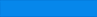# Middle School Math III Fall Semester Syllabus

• Rationalize Denominators
• Evaluating Expressions
• Divisibility
• Solving Equations
• Solving Equations with Exponents
• Solving Inequalities
• Venn Diagram
• Combinatorics
• Circular Permutation
• Probability
• Angle and Triangles
• Similar Triangles
• Pythagorean Theorem
• Trapezoid

# Middle School Math III Spring Semester Syllabus

• Functions and Operations
• Inversion Function
• Exponential Functions
• Logarithmic Functions
• Factors
• Prime numbers
• LCM and GCF
• Method of Factoring
• Venn Diagram
• Congruences
• Last digits
• Remainder
• Euler's Theorem
• Trigonometry

# Middle School Math III Summer Semester Syllabus

• Angle and Triangles
• Pythagorean Theorem
• Rectangles and Squares
• Similarity
• Circles
• 3D Geometry